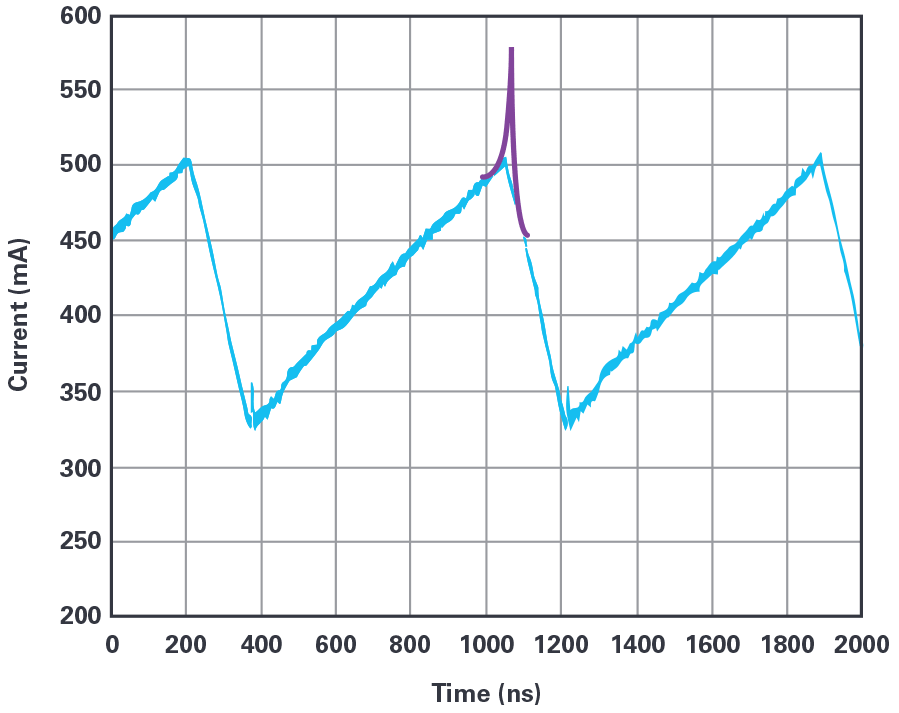# Inductor Current Measurement in Switched Power Supplies

### Вопрос:

How do you measure inductor current?

### Ответ:

Switched-mode power supplies commonly use inductors for temporarily storing energy. In the evaluation of these power supplies, it is often useful to measure the inductor current to gain a complete picture of the voltage conversion circuit. But what is the best way to measure the inductor currents?

Figure 1 shows a suggested setup for such a measurement using the example of a typical step-down converter (buck topology). A small auxiliary cable is inserted in series with the inductor. It is used to attach a current probe and display the inductor current with an oscilloscope. It is recommended that measurements be made on the side of the inductor where the voltage is stable. Most switching regulator topologies use the inductor in such a way that the voltage on one side is switched between two extreme values but remains relatively stable on the other side. With the buck converter shown in Figure 1, the voltage at the switching node—that is, the left side of inductor L—switches between the input voltage and the ground voltage at the speed of the switching edges. On the right side of the inductor is the output voltage, which is usually relatively steady. To reduce the interference due to capacitive coupling (electric field coupling), the current measurement loop should be placed on the quiet side of the inductor, as shown in Figure 1.Figure 1. Schematic illustrating measurement of the inductor current in a switched mode power supply.

Figure 2 shows the practical setup for this measurement. The inductor is lifted and obliquely soldered back with one of the two terminals on the board. The alternate terminal is connected to the board with the auxiliary wire. This conversion can be accomplished quite easily. Desoldering with hot air is a proven method for detaching the inductor. Many SMD rework stations offer hot air with an adjustable temperature.Figure 2. Practical setup for inductor current measurement.

Current probes are offered by manufacturers of oscilloscopes. Unfortunately, they are usually quite expensive—thus the question continually arises as to whether the inductor current could also be measured by means of a shunt resistor. This is possible in principle. However, this measurement has the disadvantage that the switching noise generated in a switched-mode power supply can easily couple into a voltage measurement via a shunt resistor. Thus, especially at the points of interest, when the inductor current changes direction, the measurement results would not be a true representation of the behavior of the inductor current.Figure 3. Inductor current measurement shown in blue and behavior of a saturated inductor added in purple.

Figure 3 shows the measurement of the inductor current (in blue) for a switched-mode power supply sensed with a current probe compatible with the oscilloscope used. In addition to the measurement shown in blue, a purple marking was added, indicating how the current flow through the inductor would look when the inductor starts to saturate excessively toward the peak currents. This happens when an inductor is selected that does not have enough current rating for a given application. One of the main reasons for measuring inductor current in a switched-mode power supply is so that one can recognize whether the inductor was properly selected or whether inductor saturation will occur in operation or during a fault condition.

Measurement with a shunt resistor instead of a current clamp would show strongly coupled noise, especially at the peak currents, making it difficult for inductor saturation to be detected.

Sensing of the coil current is very useful in the evaluation of a power supply and can easily be accomplished with suitable equipment.